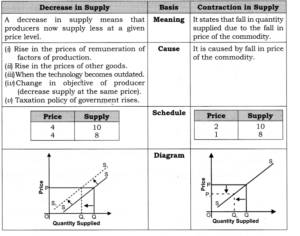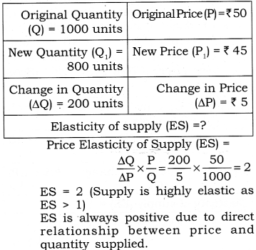# Chapter 7 – Supply Questions and Answers: NCERT Solutions for Class 12 Micro Economics

Class 12 Introduction to Economics NCERT book solutions for Chapter 7 - Supply Questions and Answers.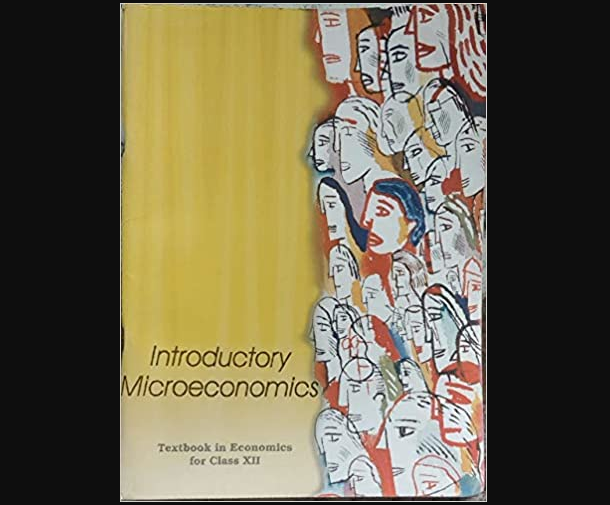## Question 1. Consider a market with two firms. The following table shows the supply schedules of two firms: the SS1 column gives supply schedule of firm 1 and SS2 column gives supply schedule of firm 2. Compute the market supply schedule.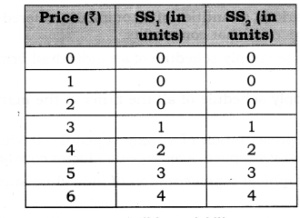## Question 2. Consider a market with two firms. In the following table, columns labelled as SS1 and SS2 give the supply schedules of firm 1 and firm 2 respectively. Compute the market supply schedule.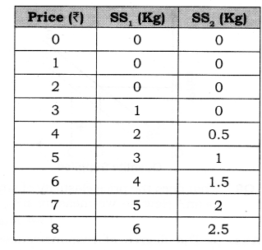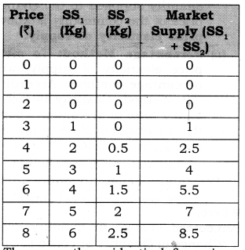## Question 3. There are three identical firms in a market. The following table shows the supply schedule of firm 1. Compute the market supply schedule.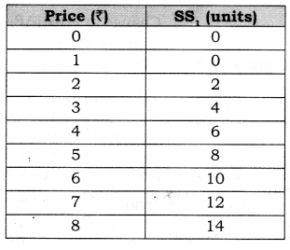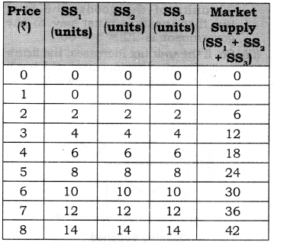## Question 4. How does technological progress affect the supply curve of a firm?

### Answer: When there is technological progress in the firm, then cost of production will decrease, which leads to increase in the profit margin of the firm and thereby shifts the supply curve rightward as showm.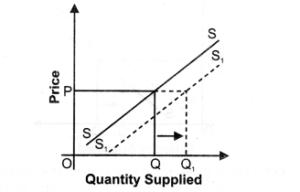## Question 5. How does the imposition of a unit tax affect the supply curve of a firm?

### Answer: (i) A unit tax is a tax that the government imposes per unit sale of output. (ii) For example, suppose that the unit tax imposed by the government is Rs 3. Then, if the firm produces and sells 20 units of the goods, the total tax that the firm must pay to the government is 20 x 3 = 60. (iii) So, if the unit tax increases, the firm’s cost of production increases which will shift the supply curve leftward.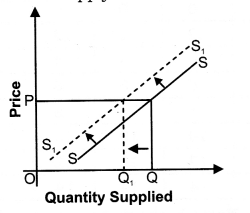## Question 6. How does an increase in price of an input affect the supply curve of a firm?

### Answer: (i) This also influences the supply since price of inputs (rent, wages, interest, profit) constitutes the cost of production of a commodity. (ii) An increase in the price of an input may lead to rise in cost of production, which will thereby decrease the production of a commodity shifting the supply curve to the left as shown.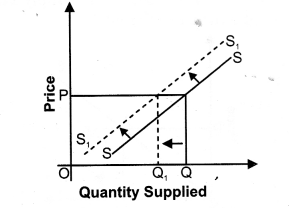## Question 7. How does an increase in the number of firms in a market affect the market supply curve?

### Answer: (i) When the number of firms in the industry increases, market supply also increases due to large number of producers producing that commodity. (ii) So, due to increase in market supply, the supply curve shifts rightward as shown.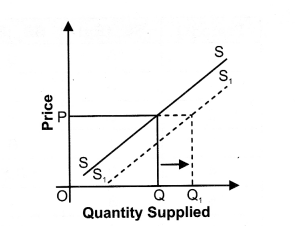## Question 8. What does the price elasticity of supply mean? How do we measure it?

### Answer: (i) The degree of responsiveness of quantity supplied to the changes in price of the commodity is known as price elasticity of supply (ii) Percentage Method: To measure price elasticity of supply, we use percentage method. According to this method, elasticity is measured as the ratio of percentage change in the quantity supplied to percentage change in the price.Price elasticity of supply (ES) Percentage change in quantity supplied Percentage change in price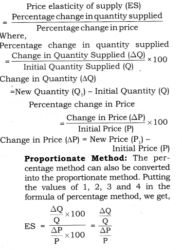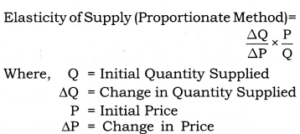## Question 9. What is the supply curve of a firm in the short run?

### (ii) The supply curve during short period is inelastic, ie., percentage change in quantity supplied is less than percentage change in price as shown below: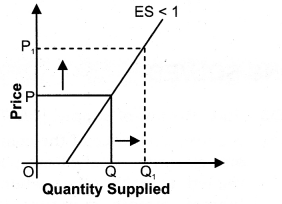## Question 10. What is the supply curve of a firm in the Long run?

#### Answer: (i) In the long period, supply is more elastic as all the factors can be changed and supply can be easily adjusted as per changes in price. (ii) The supply curve during long period is elastic, i.e., percentage change in quantity supplied is greater than per¬centage change in price as shown below: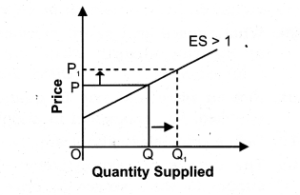## Question 11. At the market price of Rs. 10, a firm supplies 4 units of output. The market price increases to Rs. 30. The price elasticity of the firm’s supply is 1.25. What quantity will the firm supply at the new price?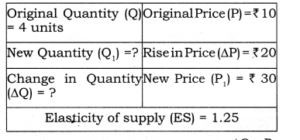## Question 12. The market price of a good changes from Rs. 5 to Rs. 20. As a result, the quantity supplied by a firm increases by 15 units. The price elasticity of the firm’s supply curve is 0.5. Find the initial and final output levels of the firm.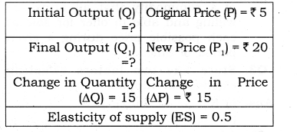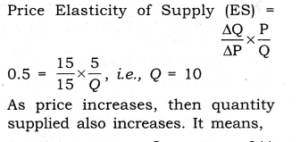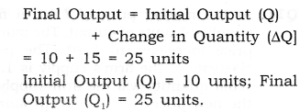## Question 13. A firm earns a revenue of Rs. 50 when the market price of a good is Rs. 10. The market price increases to Rs. 15 and the firm now earns a revenue of Rs. 150. What is the price elasticity of the firm’s supply curve?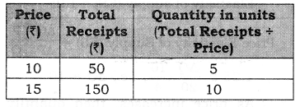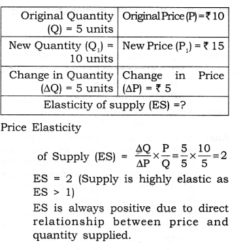## Question 1. Explain the concept of law of supply. Or State law of supply. Or What is meant by the assumption, ‘other things remaining the same’ on which law of supply is based?

### Answer: It is based on certain assumptions. If these assumptions fulfil in the economy, Law of supply states that positive relationship exists between price of the commodity and quantity supplied of that commodity.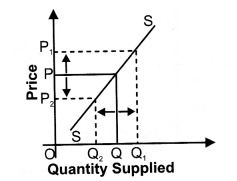### “Other things being constant (ceteris paribus), based on the price of the commodity” is called Law of Supply. It means due to rise in price of a commodity its quantity supplied also rises and vice-versa. The assumptions are as under: (a) Price of other commodity remains constant. (b) Technology of production should not change. (c) Cost of production remains constant. (d) Goal of the firm remains constant. (e) Taxation policy of the government should not change.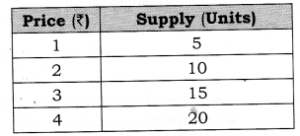## Question 2. Under what condition, a producer would like to supply more at a given level of price? Or What is increase in supply? Explain three causes of increase in supply. Or State factors that can cause a rightward shift of supply curve.

### Answer: An increase in supply means that producers now supply more at a given price level. The conditions or causes are: (a) Fall in the prices of other goods. (b) Fall in the prices of remuneration of factors of production. (c) Improvement in Technology. (d) Change in objective of producer (increase the supply at the same rate). (e) Taxation policy of government falls.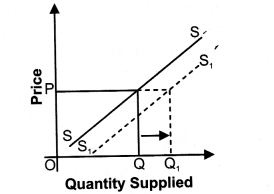## Question 3. Under what conditions, a producer would like to supply less at a given price? Or Explain only two causes of decrease in supply of a commodity. State factors for leftward shift of supply curve.

### Answer: A decrease in supply means that producers now supply less at a given price level. The conditions are: (a) Rise in the prices of remuneration of factors of production. (b) Rise in the prices of other goods. (c) When the technology becomes outdated. (d) Change in the objective of producer (decrease supply at the same price). (e) Taxation policy of government rises.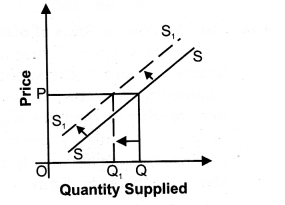## Question 4. Explain how changes in prices of inputs influence the supply of a product.

### Answer: Case I— When price of input rises: Due to rise in price of input the cost of production of a firm increases, which will thereby decrease the supply curve to the left as shown in the given figure.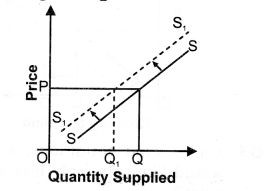### Case II— When price of input falls: Due to fall in price of input, the cost of production of a firm decreases which will thereby increase the supply curve to the right as shown in the given figure.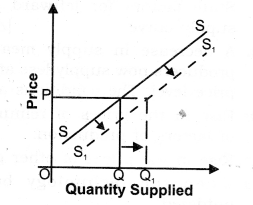## Question 5. Explain effect of ‘change in prices’ of other products on supply of a given product.

### Case II— Fall in price of Other goods: As, against it, if there is fall in price of other product, the supply curve of a given commodity increases and shifting the supply curve to the right as shown below: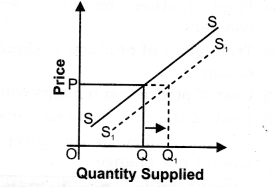## Question 6. Explain effect of technological changes on supply of a product.

### Answer: Case I— Technological Progress: When there is technological progress in the firm, then cost of production will decrease, which leads to increase in the profit margin of the firm and thereby shifts the supply curve shifts rightward as shown below: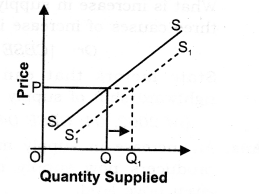## Question 7. What would be an effect on supply curve of the following: (a) Decrease in tax on product. (b) Subsidy on production of goods. (c) Rise in own price of a piece of goods.

### Answer: (a) Decrease in tax on product: Due to decreasein tax on product the cost of production of a firm decreases and supply of a given commodity increases and thereby shifts the supply curve rightward as shown in the given figure: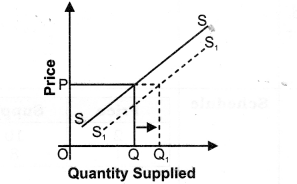### (b) Subsidy on production of goods: If a government gives subsidy to a firm for production of goods, the cost of production of firm decreases for producing this goods, that will increase the supply and thereby shifting in the supply curve to the right as shown in the given figure: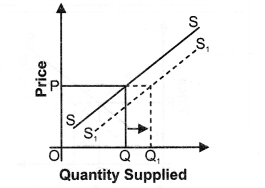### (c) Rise in own price of a good: Due to rise in own price of a good, the quantity supplied for a given commodity also rises because there is positive relationship between own price of a good and quantity supplied of a given commodity. So, due to rise in price of a commodity, there will be upward movement along the supply curve as given below: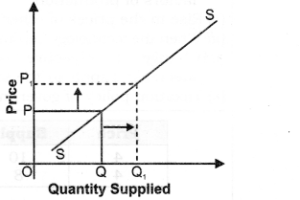## Question 8. Differentiate between increase in supply quantity supplied and expansion in supply increase in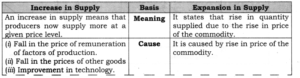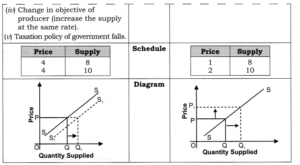## Question 9. Differentiate between decrease in supply and contraction in supply (decrease in quantity supplied).